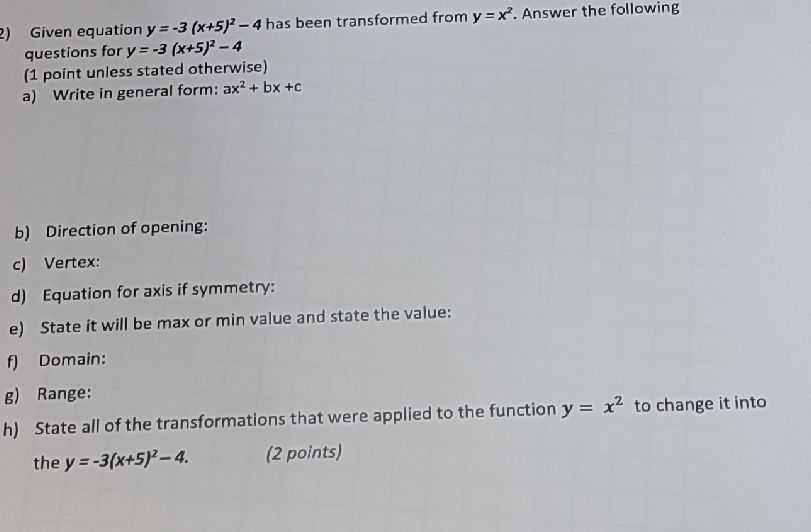### ¿Todavía tienes preguntas de matemáticas?

Pregunte a nuestros tutores expertos
Algebra
Pregunta2) Given equation $$y = - 3 ( x + 5 ) ^ { 2 } - 4$$ has been transformed from $$y = x ^ { 2 }$$ . Answer the following questions for $$y = - 3 ( x + 5 ) ^ { 2 } - 4$$

$$1$$ point unless stated otherwise)

a) Write in general form: $$a x ^ { 2 } + b x + c$$

b) Direction of opening:

c) Vertex:

d) Equation for axis if symmetry:

e) State it will be max or min value and state the value:

f) Domain:

g) Range:

h) State all of the transformations that were applied to the function $$y = x ^ { 2 }$$ to change it into the $$y = - 3 ( x + 5 ) ^ { 2 } - 4$$ .

$$a)y= -3(x+ 5)^2- 4= -3(x^2+ 10x+ 25)- 4= -3x^2- 30x- 79\\b)downward\\c)(- 5,- 4)\\d)x= -5\\e)max: -4\\f)R\\g)(- \infty ,- 4]\\$$